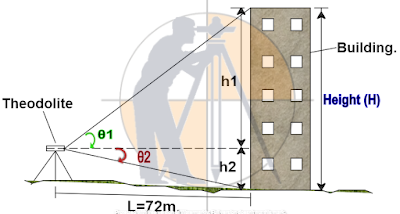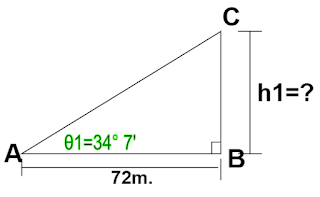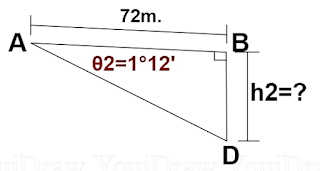Breaking News
Home / Land Surveying / How To Calculate The Height Of Any Buildings And An Objects Using With Theodolite

# How To Calculate The Height Of Any Buildings And An Objects Using With Theodolite

## How To Calculate The Height Of Any Buildings And An Objects Using With Theodolite

Let us now calculate the height of the building using a theodolite as shown below.First, you have to level the theodolite in the vertical axis and set the angle to 0° in the horizontal axis i.e. shown as a dotted line. You have to measure the distance (L ) of the building from the theodolite station point as shown in the drawing.

Bisect the top edge corner of the building using the theodolite and note down the value of angle θ1. Similarly, bisect the bottom edge corner of the building and write down the value of angle θ2.

Let us redraw the above triangles for the calculation purpose with the measured angle values.

Let us name triangle 1 as ABC, where A is the theodolite station point.

### How To Find The Coordinates Of  Point P With The Help Of Point A & B

Triangle ABC :Given data:

Angle θ1 = 34° 7′

Length AB = L = 72m.

From trigonometry,

Tan θ1

= opposite side ÷ adjacent side.

= side BC ÷ side AB

Tan 34° 7′

= h1 ÷ 72m.

h1 =  tan 34° 7′ × 72m.

= 0.67747 × 72m

48.778m.

Let us name triangle 2 as ABD, where A is the theodolite station point.

### Triangle ABD.### Given data:

Angle θ2 = 1° 12′

Length AB = L = 72m.

From trigonometry,

Tan θ2 =

= side BD ÷ side AB

Tan 1° 12′

= h2 ÷ 72m.

h2 =  tan 1° 12′ × 72m.

= 0.02094 × 72m

1.508m.

### Now, the height of building

H = h1 + h2

= 48.778m. + 1.508m.

= 50.286m.

### THANKS.